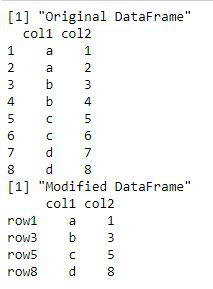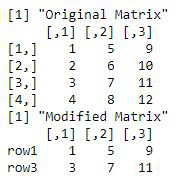GFG App
Open AppBrowser
Continue

# Subset DataFrame and Matrix by Row Names in R

In this article, we are going to see how to evaluate subset dataframe and matrices by row name.

## Method 1: Subset dataframe by row names

The rownames(df) method in R is used to set the names for rows of the data frame. A vector of the required row names is specified. The %in% operator in R is used to check for the presence of the data frame row names in the vector of required row names. The rows of the data frame are then retrieved from the data frame if they occur in the vector. The row names are returned in the final output of the data frame. The output is then used to return a subset of the data frame rows.

Syntax: val %in% vec

Arguments :

• val – A list or vector of values to check in vector
• vec – A vector to check the values in

Code:

## R

 `# creating data frame ` `data_frame <- ``data.frame``(col1 = ``rep``(``letters``[1:4], each = 2), ` `                         ``col2 = 1:8 ` `                         ``) ` `print``(``"Original DataFrame"``) ` `print``(data_frame) ` ` `  `# assigning row names to data frame ` `rownames``(data_frame) <- ``c``(``"row1"``,``"row2"``,``"row3"``,``"row4"``, ` `                          ``"row5"``, ``"row6"``,``"row7"``,``"row8"``) ` ` `  `# getting rows  ` `rows <- ``c``(``"row1"``,``"row3"``,``"row5"``,``"row8"``) ` ` `  `# extracting data frame rows ` `data_mod <- data_frame[``rownames``(data_frame) %``in``% rows, ]  ` `print``(``"Modified DataFrame"``) ` `print``(data_mod)`

Output:## Method 2: Subset matrix by row names

The rownames(mat)method in R is used to set the names for rows of the matrix. A similar approach is used to check for the presence of row names of the matrix in the vector or list of specified row names. The following code snippet can be used to subset the matrix based on the specified row names.

Code:

## R

 `# creating matrix ` `matr <- ``matrix``(1:12, nrow = 4) ` `print``(``"Original Matrix"``) ` `print``(matr) ` ` `  `# assigning row names to data frame ` `rownames``(matr) <- ``c``(``"row1"``,``"row2"``,``"row3"``,``"row4"``) ` `                           `  `# getting rows  ` `rows <- ``c``(``"row1"``,``"row3"``) ` ` `  `# extracting data frame rows ` `data_mod <- matr [``rownames``(matr) %``in``% rows, ]  ` `print``(``"Modified Matrix"``) ` `print``(data_mod)`

Output:My Personal Notes arrow_drop_up